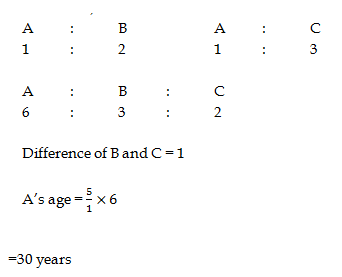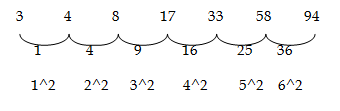# RRB ALP 2018 Practice Test Papers | Arithmetic Questions (Day-75)

Dear Aspirants, Here we have given the Important RRB ALP & Technicians Exam 2018 Practice Test Papers. Candidates those who are preparing for RRB ALP 2018 can practice these Arithmetic Questions to get more confidence to Crack RRB 2018 Examination.

[WpProQuiz 3040]

Click “Start Quiz” to attend these Questions and view Solutions

1) If P381 is divisible by 11, then the digit at the place of P is

(a) 0

(b) 1

(c) 4

(d) 7

2­) A’s age is twice that of B’s Age and Thrice that of C’s age. The difference between ages of B and C is 5, and then what is the age of A?

a) 30

b) 35

c) 40

d) 45

3) Simplify:

5.05 + 8.999 – 14.666 + 3.2121 =?

a) 0

b) 1

c) 2

d) 3

4) A Shop keeper allows a discount of 10% on marked price of his product in the way that he earned 20% profit. Find the possible cost price of his product?

a) 150

b) 125

c) 100

d) 75

5) 32% 0f 50 + 67% of 700 =?

a) 475

b) 470

c) 485

d) 480

6) Find the missing value of given series

3, 4, 8, 17, 125, ?, 194

a) 98

b) 96

c) 58

d) 48

7) A man lends a part of certain amount with the interest rate on 10% and rest of part lends with 20% for simple interest. After 2 years he received 132 as interest for that whole amount. What is the whole amount he lends?

a) 36000

b) 24000

c) 12000

d) Can’t be determined

8) LCM of two numbers is 225 and their HCF is 5. If one number is 25, the other number will be:

a) 5

b) 25

c) 45

d) 2253

9) Three bells ring simultaneously at 11 a.m. They ring at regular intervals of 20 minutes, 30 minutes, and 40 minutes respectively. The time when all the three ring together next is :

а) 2 р.m.

b) 1 p.m.

c) 1.15 p.m.

d) 2.30 p.m.

10) Ganesh can do a piece of work in 3 days and Mahesh can do the work in 2 days. With the help of Avinash they finish the work in a day. Avinash alone complete the work in how many days?

a) 4

b) 3

c) 6

d) 5

Divisible Rule for 11 is Difference of alternative numbers is 0 or 11

P381

(P+8) – (3+1) = 0 => P+8 – 4 = 0 => P = -4 (The number does not a negative value)

(P +8) – (3+1) = 11 = P + 8 – 4 = 11

P + 4 = 11 => P = 75+9-15+3 = 17 – 15 = 2

Let marked price = 100

After giving 10% discount

Selling price = 90

Cost price = = 75

32% 0f 50 + 67% of 700 = 469 + 16 = 485.HCF×LCM = 1st number × 2nd Number

225×5 = 25 × X

X = (225×5)/25 = 45.

LCM of 20, 30, and 40 is 120

120/60 = 2 hours

After 2 hours 1 pm all the three bells ring together.

Ganesh’s One day work = 1/3

Mahesh’s one day work = 1/2

Ganesh + Mahesh + Avinash = 1

1/3 + ½ + Avinash = 1

Avinash = 1 – 1/3 – 1/2 = 1/6

Avinash can alone do the work in 6 days

RRB ALP 2018 – “All in One” Study Materials and Practice Sets